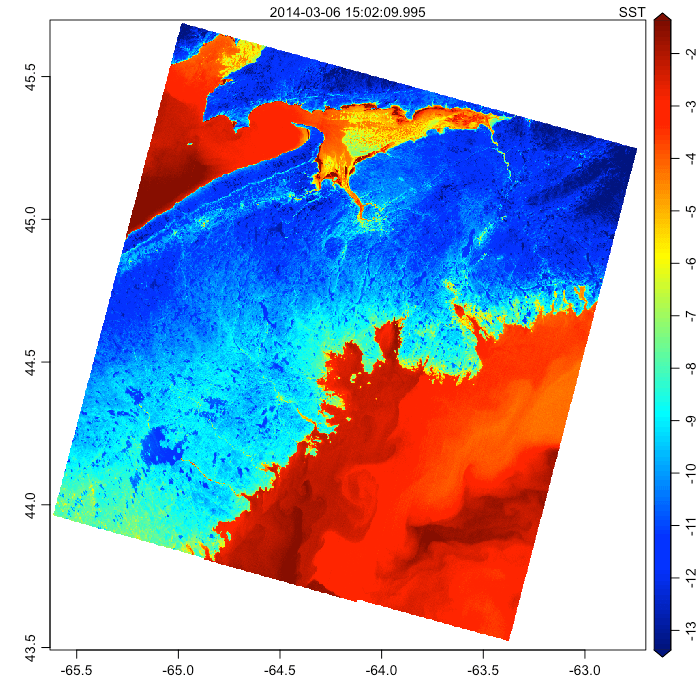Want to share your content on R-bloggers? click here if you have a blog, or here if you don't.

# Introduction

The tirs1 band of landsat-8 imagery (see ) is a near-thermal band, from which a quantity called “brightness temperature’’ may be inferred.

As explained in , the brightness temperature in Kelvin is computed with $T=K_2 / ln(K_1/\lambda_L + 1)$ where $K_1$ and $K_2$ are stored in the image metadata, and $\lambda_L=M_L*d+A_L$, where again $M_L$ and $A_L$ are stored in the metadata. In this last expression, $d$ is the image value, which when read with the oce package must be multiplied by a scale factor for the formulae to work.

# Methods

Note in the code that the SST is computed in Celcius, and inserted into the data object before plotting.

  1 2 3 4 5 6 7 8 9 10 11 12 13 14 library(oce) l <- read.landsat("LC80080292014065LGN00", band="tirs1") tirs1 <- l[["tirs1"]] ML <- [email protected]$header$radiance_mult_band_10 AL <- [email protected]$header$radiance_add_band_10 K1 <- [email protected]$header$k1_constant_band_10 K2 <- [email protected]$header$k2_constant_band_10 d <- tirs1 * (2^16 - 1) # convert from range 0 to 1 Llambda <- ML * d + AL dd <- K2 / log(K1 / Llambda + 1) SST <- dd - 273.15 # convert Kelvin to Celcius [email protected]\$SST <- SST plot(l, band="SST", col=oceColorsJet) mtext(l[["time"]]) 

# Results

The figure below shows the results. This image is from winter, so the land (Nova Scotia) is colder than the ocean.

The high land is especially cold, as those familiar with the topography can tell at a glance. Homogenous blue patches indicate frozen lakes.

Generally, the ocean temperature hovers around freezing (i.e. -2C). The anomalously cold patches in the Bay of Fundy are probably a signature of ice. Some of the orange colour suggests temperatures a much as 1C below freezing. Whether this indicates a difficulty in the algorithm or the interpretation is a matter for Landsat experts.

The beautiful swirls south of Nova Scotia are typical of these coastal waters.# Resources

1. Landsat bands are explained at http://landsat.gsfc.nasa.gov/?page_id=5377.

2. The conversion to brightness temperature is described at http://landsat.usgs.gov/Landsat8_Using_Product.php

3. Source code: 2014-07-01-landsat.R.